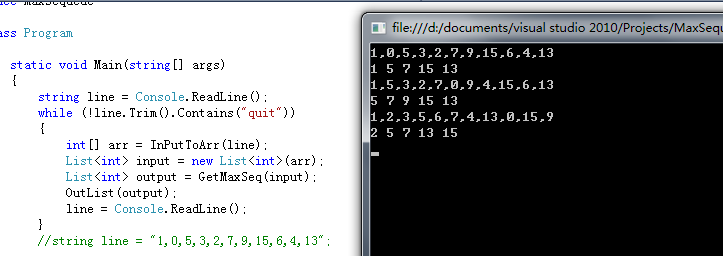9个回答

``````public static List<Integer> longest_increasing_subsequence(List<Integer> sequence) {
if (sequence.isEmpty()) return new ArrayList<Integer>();
int max_size[] = new int[sequence.size()];
int prev_index[] = new int[sequence.size()];
max_size = 1;
prev_index = -1;
for (int i = 1; i < sequence.size(); i++) {
int cur_max = 1;
int cur_prev = -1;
int iVal = sequence.get(i);
for (int j = i - 1; j >= 0; j--) {
if (cur_max < max_size[j] + 1 && sequence.get(j) < iVal) {
cur_max = max_size[j] + 1;
cur_prev = j;
}
}
prev_index[i] = cur_prev;
max_size[i] = cur_max;
}
int max_idx = sequence.size() - 1;
int max_val = max_size[max_idx];
for (int i = max_idx - 1; i >= 0; i--) {
if (max_val < max_size[i]) {
max_idx = i;
max_val = max_size[i];
}
}
List<Integer> aList = new ArrayList<Integer>();
int idx = max_idx;
while (prev_index[idx] >= 0) {
idx = prev_index[idx];
}
List<Integer> retList = new ArrayList<Integer>(aList.size());
for (int i = aList.size() - 1; i >= 0; i--) {
}
return retList;
}
``````
``````public static String[] getMaxSubArray(int[] arr) {
String[] strArr = new String[arr.length];
for(int i =0;i<arr.length;i++) {
strArr[i] = String.valueOf(arr[i]);
}
for(int i=2;i<arr.length;i++) {
if (arr[i-2] + arr[i] > arr[i-1]) {
arr[i] = arr[i-2] + arr[i];
strArr[i] = strArr[i-2]+","+strArr[i];
} else {
arr[i] = arr[i-1];
strArr[i] = strArr[i-1];
}
}
return strArr[strArr.length-1].split(",");
}

``````weixin_42042460 回复flonny: 这个能用分治来做吗weixin_42042460 你的结果是2，8 正确的应该是5，8weixin_42042460 arr={5,2,1,8}weixin_42042460 这个可以的，可以把字符串数组换成list来存吗？``````private static boolean isValueNotNextInArray(List<Integer> sequence, int ival, int cur_prev, int[] prev_index) {
while(cur_prev >= 0) {
int prev_val = sequence.get(cur_prev);
if (Math.abs(ival - prev_val) == 1) return false;
cur_prev = prev_index[cur_prev];
}
return true;
}

public static List<Integer> longest_notnext_subsequence(List<Integer> sequence) {
if (sequence.isEmpty()) return new ArrayList<Integer>();
int max_size[] = new int[sequence.size()];
int prev_index[] = new int[sequence.size()];
max_size = 1;
prev_index = -1;
for (int i = 1; i < sequence.size(); i++) {
int cur_max = 1;
int cur_prev = -1;
int iVal = sequence.get(i);
for (int j = i - 1; j >= 0; j--) {
if (cur_max < max_size[j] + 1 && isValueNotNextInArray(sequence, iVal, j, prev_index)) {
cur_max = max_size[j] + 1;
cur_prev = j;
}
}
prev_index[i] = cur_prev;
max_size[i] = cur_max;
}
int max_idx = sequence.size() - 1;
int max_val = max_size[max_idx];
for (int i = max_idx - 1; i >= 0; i--) {
if (max_val < max_size[i]) {
max_idx = i;
max_val = max_size[i];
}
}
List<Integer> aList = new ArrayList<Integer>();
int idx = max_idx;
while (prev_index[idx] >= 0) {
idx = prev_index[idx];
}
List<Integer> retList = new ArrayList<Integer>(aList.size());
for (int i = aList.size() - 1; i >= 0; i--) {
}
return retList;
}
``````

public static void testLongArray() {
List vals = longest_notnext_subsequence(Arrays.asList(1, 0, 5, 3, 2, 7, 9, 15, 6, 4, 13));
System.out.println(vals);
}

public static void main(String args[]) {
testLongArray();
}

``````结果是：
[0, 5, 2, 7, 9, 15, 13]

``````

static List GetMaxSeq(List list)
{
List res = new List();
List ou = new List();
List qi = new List();
for (int i = 0; i < list.Count; i++)
{
if (i%2==0) //奇偶分裂，奇偶数列都是连续不相邻的
{
}
else
{
}
}
int last = 0;
for (int i = 0; i < qi.Count; i++)
{
if (ou[i] {
ou[i] = qi[i]; // 在相等位置上将奇列中大于偶列中的数替换到偶列
last = i;
}
}
for (int i = 0; i {
if (i!=last+1) // 记录最后的替换位置，防止替换后结果连续，那么即排除奇最后位+1的偶数，在原数列奇偶相等时还需判断是否超索引
{
}
}
return res;
}
static void OutList(List list)
{
string res = "";
foreach (T item in list)
{
res += item + " ";
}
Console.WriteLine(res);
}
static int[] InPutToArr(string line)
{
string[] nums=line.Split(',');
int[] res=new int[nums.Length ];
for (int i = 0; i < nums.Length; i++)
{
res[i] = int.Parse(nums[i]);
}
return res;
}
static void Main(string[] args)
{
while (!line.Trim().Contains("quit"))
{
int[] arr = InPutToArr(line);
List input = new List(arr);
List output = GetMaxSeq(input);
OutList(output);
}
//string line = "1,0,5,3,2,7,9,15,6,4,13";

}weixin_42042460 回复weixin_39521929: 我会再开一个问题，你的qq多少weixin_42042460 代码有的地方漏了吧，能发个有格式的嘛

`````` public static Integer[] getMaxSubArray(int[] arr) {
List<Integer> list = new ArrayList<Integer>();
int index = 0;
do {
if(index+2 >= arr.length)
break;
if(index+2 < arr.length && index+3 >= arr.length) {
break;
}
if(arr[index+2] >= arr[index+3])
index += 2;
else
index += 3;
} while(index<arr.length);

Integer[] resultArray = new Integer[list.size()];
list.toArray(resultArray);
return resultArray;
}
``````weixin_42042460 回复flonny: 问题就是dp只能求出最大和，不能求出每个数字把weixin_42042460 0,100,5,3,2,7,9,15这个就错了，你这个是首先把第一项加进去吧，如果第二项比第一个大呢？

public static List longest_maxval_subsequence(List sequence) {
if (sequence.isEmpty()) return new ArrayList();
int max_vals[] = new int[sequence.size()];
int prev_index[] = new int[sequence.size()];
max_vals = sequence.get(0);
prev_index = -1;
for (int i = 1; i < sequence.size(); i++) {
int cur_max = sequence.get(i);
int cur_prev = -1;
int iVal = sequence.get(i);
for (int j = i - 2; j >= 0; j--) {
if (cur_max < max_vals[j] + iVal) {
cur_max = max_vals[j] + iVal;
cur_prev = j;
}
}
prev_index[i] = cur_prev;
max_vals[i] = cur_max;
}
int max_idx = sequence.size() - 1;
int max_val = max_vals[max_idx];
for (int i = max_idx - 1; i >= 0; i--) {
if (max_val < max_vals[i]) {
max_idx = i;
max_val = max_vals[i];
}
}
List aList = new ArrayList();
int idx = max_idx;
while (prev_index[idx] >= 0) {
idx = prev_index[idx];
}
List retList = new ArrayList(aList.size());
for (int i = aList.size() - 1; i >= 0; i--) {
}
return retList;
}weixin_42042460 你这个是分治法？``````public static String[] getMaxSubArray(int[] arr) {
String[] strArr = new String[arr.length];
for(int i =0;i<arr.length;i++) {
strArr[i] = String.valueOf(arr[i]);
}
for(int i=1;i<arr.length;i++) {
if(i<2) {
if (arr[i-1] > arr[i]) {
arr[i] = arr[i - 1];
strArr[i] = strArr[i-1];
}
continue;
}
if (arr[i-2] + arr[i] > arr[i-1]) {
arr[i] = arr[i-2] + arr[i];
strArr[i] = strArr[i-2]+","+strArr[i];
} else {
arr[i] = arr[i-1];
strArr[i] = strArr[i-1];
}
}
return strArr[strArr.length-1].split(",");
}

``````-

-

-

-
c语言编译的最大子序列求和问题
-
java 求最大子序列和问题递归求解报越界异常
-

-

-

-
Oracle有关取序列问题
-

-

-
A sequence of numbers 序列的问题
-
Java实现：最长上升子序列LIS算法
-

-

-

-

-

-

996下的程序员，该如何保证自己的身体健康？

Spring高级技术梳理
Spring高级技术梳理 序言正文SpringDate部分Spring全家桶之SpringData——预科阶段Spring全家桶之SpringData——Spring 整合Hibernate与Hibernate JpaSpring全家桶之SpringData——Spring Data JPASpring全家桶之SpringData——SpringData RedisSpringBoot部分Sp...
Git 天天用 但是 Git 原理你了解吗？
Git 原理 做技术一定要知其然知其所以然，意思就是：知道它是这样的，更知道它为什么是这样的。我主要通过4块内容来简单介绍 Git 是原理是什么样的。这4块内容如下： Git 存储目录结构介绍 Git 是如何存储的 Git 的对象 Git引用 当然 Git 原理不仅仅包含这些，想要更深入了解请查看官方教程 https://git-scm.com/book/zh/v2/。 本文内容是我在 Git...
Android——微信自动回复实现

8000字干货：那些很厉害的人是怎么构建知识体系的

Java 网络爬虫，就是这么的简单

nginx学习，看这一篇就够了：下载、安装。使用：正向代理、反向代理、负载均衡。常用命令和配置文件

Java 爬虫遇上数据异步加载，试试这两种办法！

Angular 入门教程系列：39：使用ng-alain进行开发